CBSE Class 10 Sample Paper for 2024 Boards - Maths Standard

Class 10
Solutions of Sample Papers for Class 10 Boards

## A tent is in the shape of a cylinder surmounted by a conical top. If the height and radius of the cylindrical part are 3 m and 14 m respectively, and the total height of the tent is 13.5 m, find the area of the canvas required for making the tent, keeping a provision of 26 m^2 of canvas for stitching and wastage. Also, find the cost of the canvas to be purchased at the rate of ₹ 500 per m^2.

This question is similar to Ex 12.1, 7 – Chapter 12 Class 10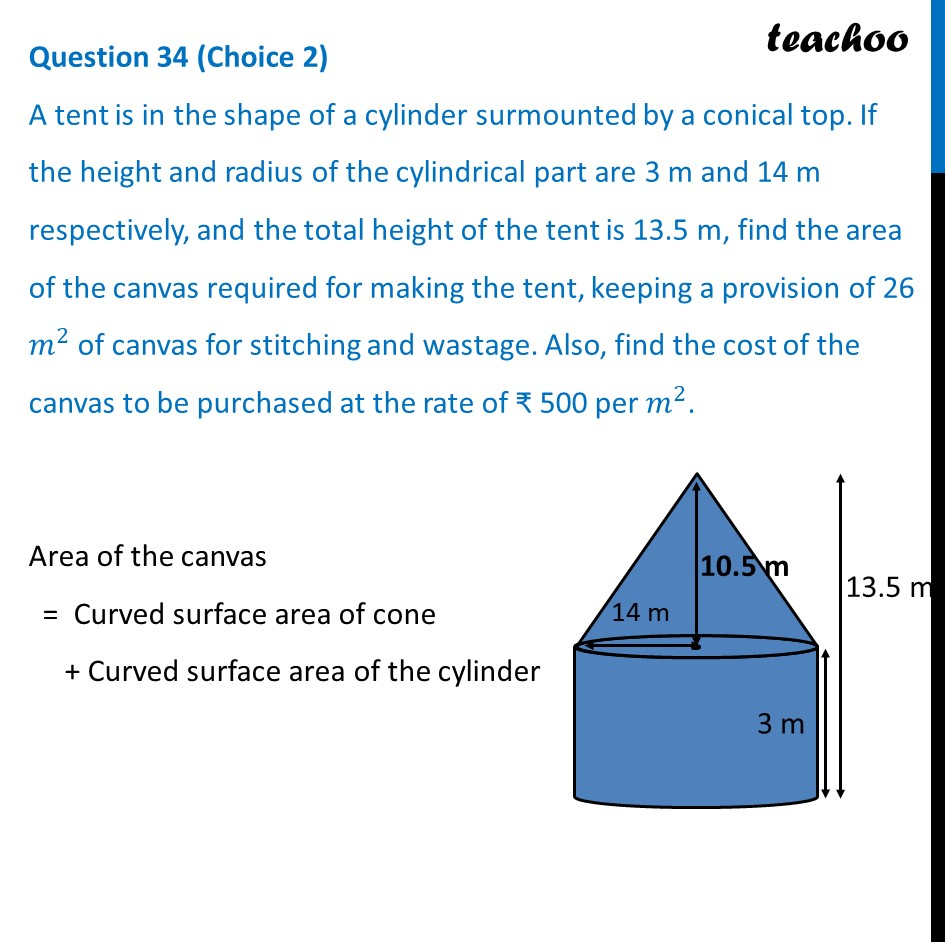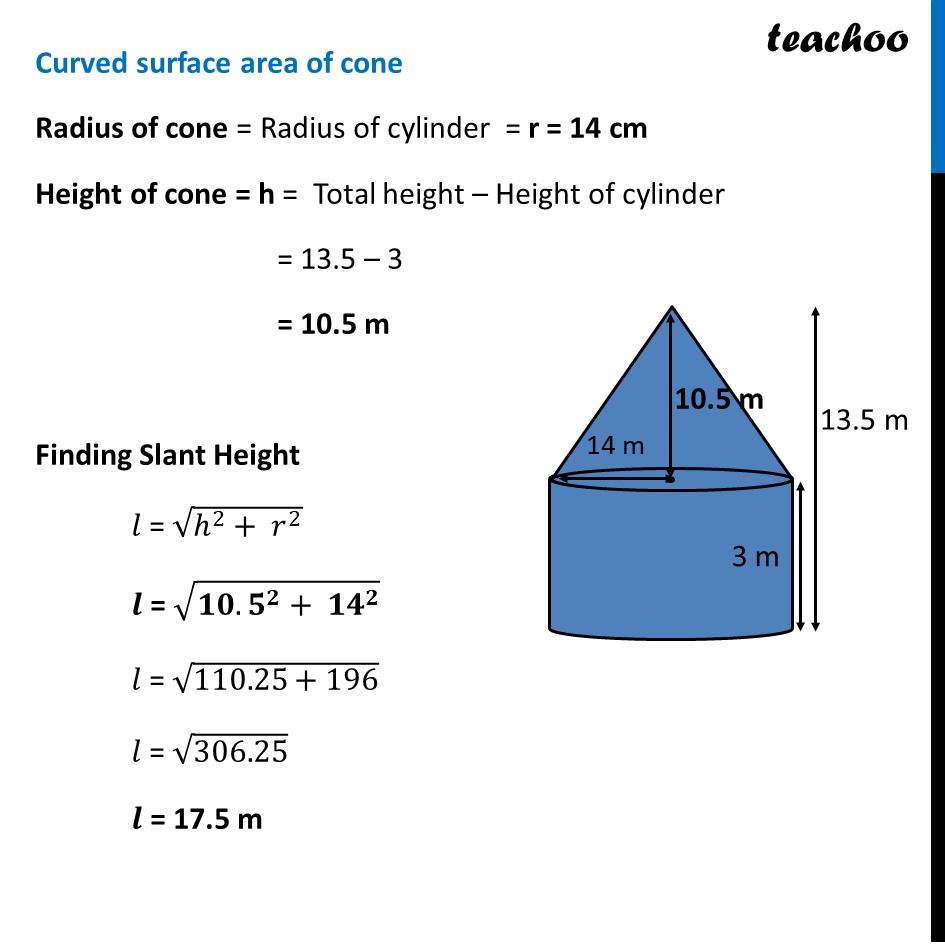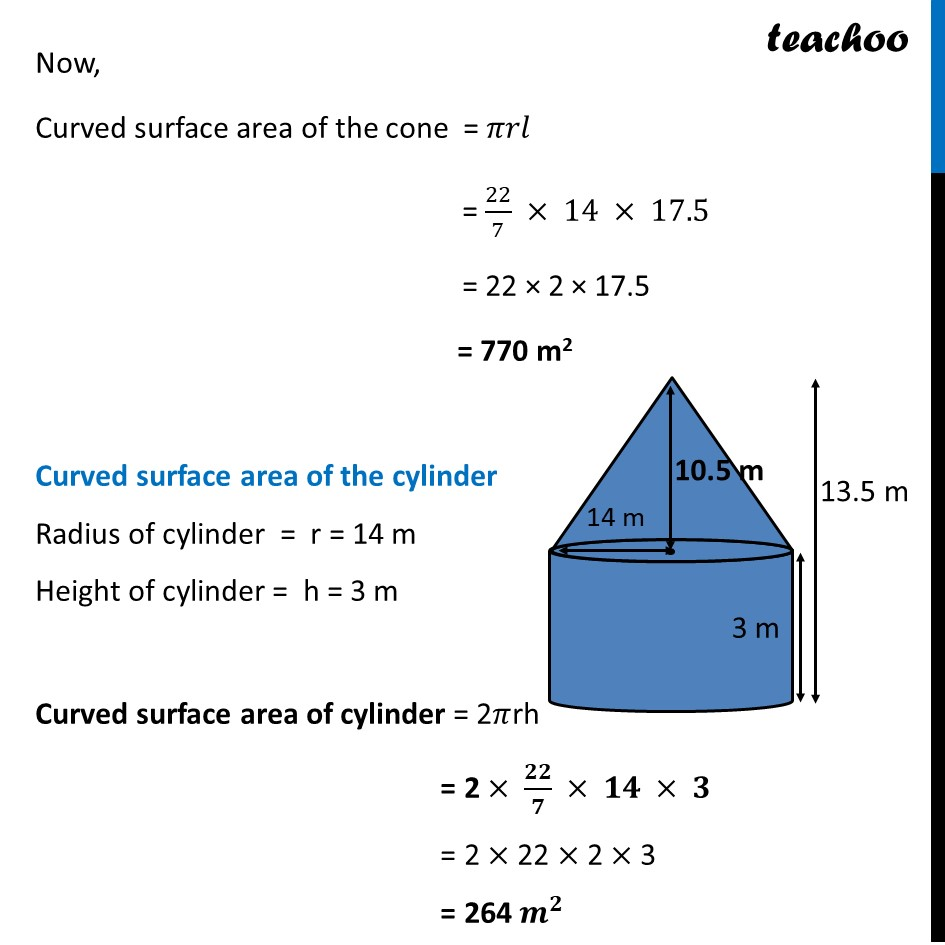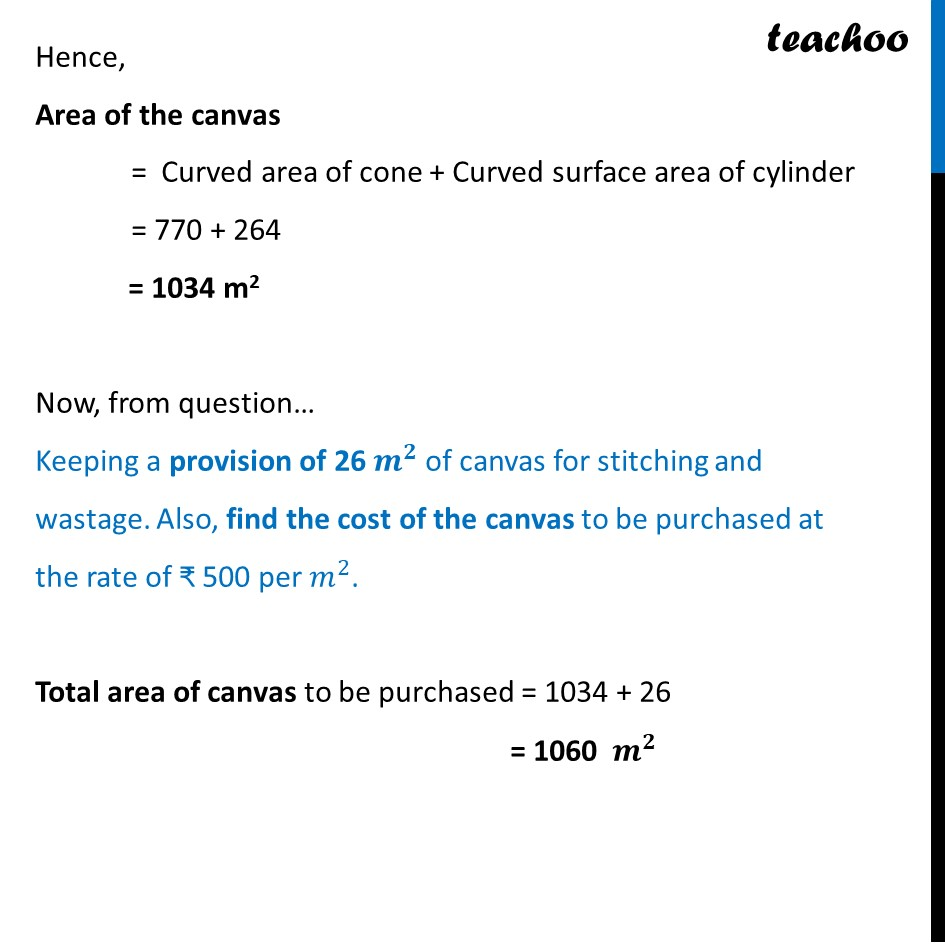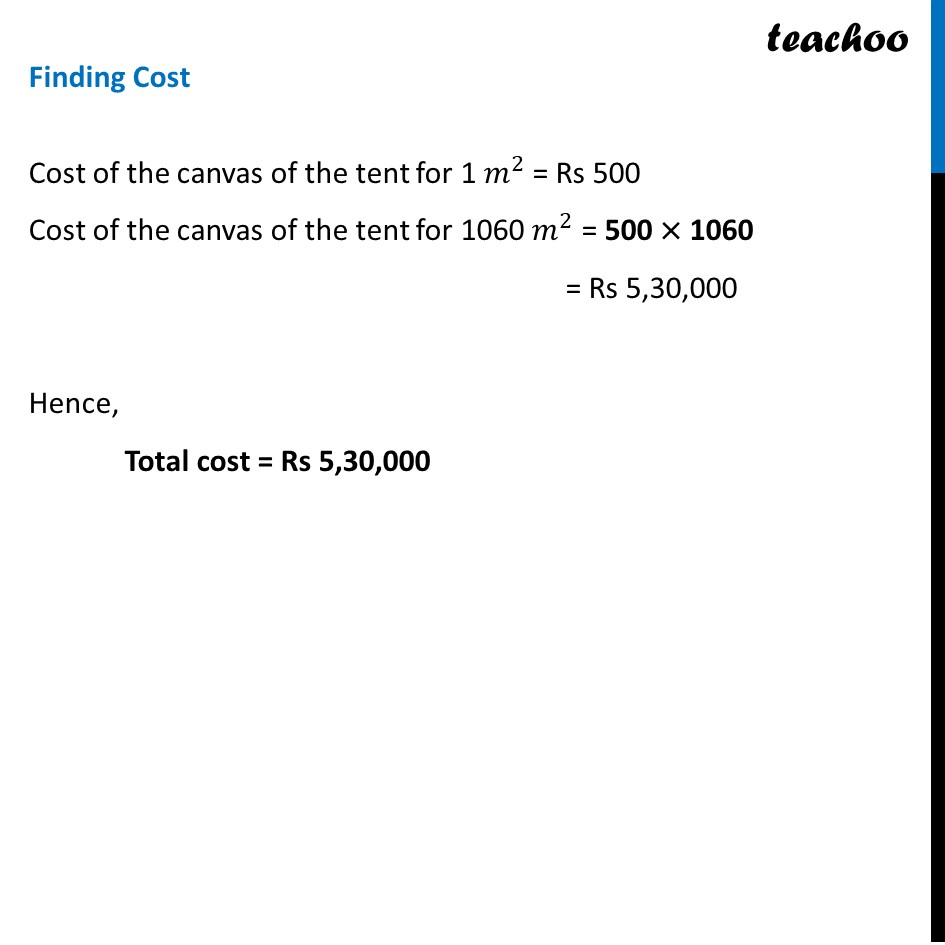Learn in your speed, with individual attention - Teachoo Maths 1-on-1 Class

### Transcript

Area of the canvas = Curved surface area of cone + Curved surface area of the cylinder Curved surface area of cone Radius of cone = Radius of cylinder = r = 14 cm Height of cone = h = Total height – Height of cylinder = 13.5 – 3 = 10.5 m Finding Slant Height 𝑙 = √(ℎ^2+ 𝑟^2 ) 𝒍 = √(〖𝟏𝟎.𝟓〗^𝟐+ 〖𝟏𝟒〗^𝟐 ) 𝑙 = √(110.25+196) 𝑙 = √306.25 𝒍 = 17.5 m Now, Curved surface area of the cone = 𝜋𝑟𝑙 = 22/7 × 14 × 17.5 = 22 × 2 × 17.5 = 770 m2 Curved surface area of the cylinder Radius of cylinder = r = 14 m Height of cylinder = h = 3 m Curved surface area of cylinder = 2𝜋rh = 2 × 𝟐𝟐/𝟕 × 𝟏𝟒 × 𝟑 = 2 × 22 × 2 × 3 = 264〖 𝒎〗^𝟐 Hence, Area of the canvas = Curved area of cone + Curved surface area of cylinder = 770 + 264 = 1034 m2 Now, from question… Keeping a provision of 26 𝒎^𝟐 of canvas for stitching and wastage. Also, find the cost of the canvas to be purchased at the rate of ₹ 500 per 𝑚^2. Total area of canvas to be purchased = 1034 + 26 = 1060 〖 𝒎〗^𝟐 Finding Cost Cost of the canvas of the tent for 1〖 𝑚〗^2 = Rs 500 Cost of the canvas of the tent for 1060〖 𝑚〗^2 = 500 × 1060 = Rs 5,30,000 Hence, Total cost = Rs 5,30,000